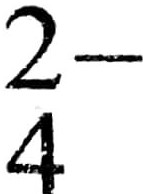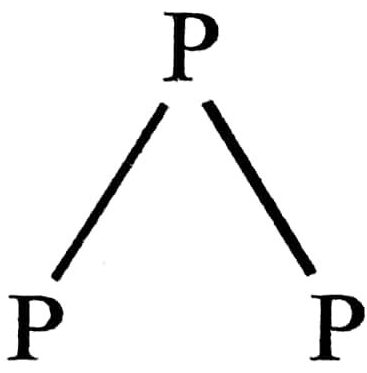7. THE P-BLOCK ELEMENTS

1. Boron shows diagonal relation with

(A) Al
(B) C
(C) Si
(D) Sn

2. Catenation property is maximum in

(A) phosphorus
(B) carbon
(C) sulphur
(D) zinc

3. Which one of the following is an electrophilic reagent ?

(A) BF3
(B) NH3
(C) H2O
(D) None of these

4. Element found from sea water is

(A) Magnesium
(B) Sodium
(C) Iodine
(D) None of these

5. The shape of XeF 4 is

(A) tetrahedral
(B) square planar
(C) pyramidal
(D) linear

6. Which one of the following is the strongest lewis acid ?

(A) BF3
(B) BC13
(C) BBr3
(D) BI3

7. Which one of the following does not form hydrogen bonding ?

(A) NH3
(B) H2O
(C) HCl
(D) HF

8. Main source of helium is

(A) Air
(C) Monazite
(D) Water

9. Which one of the following element is liquid at normal temperature ?

(A) zinc
(B) mercury
(C) bromine
(D) water

10. Which one of the following is least basic ?

(A) NCl3
(B) NBr3
(C) NI3
(D) NF3

11. H2SO4 is a/an

(A) acid
(B) base
(C) alkali
(D) salt

12. Modern periodic table is given by

(A) Debonair
(B) Mendeleef
(C) Mendel
(D) None of these

13. Which one of the following elements is found in free state in nature ?

(A) Sodium
(B) Iron
(C) Zinc
(D) Gold

14. Which of the following is the strongest oxidising agent ?

(A) F2
(B) Cl2
(C) I2
(D) Br2

15. Which of the following has maximum ionisation potential ?

(A) Al
(B) P
(C) Si
(D) Mg

16. The number of P-O-P bonds in cyclic metaphosphoric acid is

(A) 2
(B) O
(C) 3
(D) 4

17. Which of the following oxides is anhydride of nitrous acid ?

(A) N2O3
(B) NO2
(C) NO
(D) N2O4

18. Which oxide of nitrogen is obtained on heating ammonium nitrate at 250°C ?

(A) Nitric oxide
(B) Nitrous oxide
(C) Nitrogen dioxide
(D) Dinitrogen tetraoxide

19. Atomicity of phosphorus is

(A) 1
(B) 2
(C) 3
(D) 4

20. The structure of white phosphorus is

(A) square-planar
(B) pyramidal
(C) tetrahedral
(D) trigonal planar

21. How many P-O-P bonds appear in cyclic meta phosphoric acid ?

(A) 4
(B) 3
(C) 2
(D) 1

22. Which of the following is a tetrabasic acid ?

(A) Hypophosphorus acid
(B) Metaphosphoric acid
(C) Pyrophosphoric acid
(D) Orthophosphoric acid

23. Which of the following is not correctly matched ?

(A) PCl5-sp3d hybridisation
(B) PCl3-sp3 hybridisation
(C) PCl5 (solid)-[PtCl4]+ [PtC6l-
(D) H3PO3-tribasic

24. Arrange the following hydrides of group 16 elements in order of increasing stability

(A) H2S < H2O < H2 Te > H2 Se
(B) H2O < H2Te < H2Se < H2S
(C) H2O < H2S < H2Se < H2Te
(D) H2Te < H2Se < H2S < H2O

25. Which of the following is not tetrahedral in shape ?

(A) NH4
(B) SiCl4
(C) SF4
(D) SO26. Which of the following are peroxiacids of sulphur ?

(A) H2SO5 and H2S2O8
(B) H2SO5 and H2S2O7
(C) H2S2O7 and H2S2O8
(D) H2S2O6 and H2S2O8

27. In solid state PCl5 is a

(A) covalent solid
(B) octahedral structure
(C) ionic solid with [PCl6l+ octahedral and [PCl4] tetrahedral
(D) ionic solid with [PCl4]+ tetrahedral and [PCl6] octahedral

28. Which of the following is an isoelectronic pair ?

(A) ICI2, CIO2
(B) Br2, BrF2+
(C) CIO2, BrF
(D) CN, O3

29. Which of the following is Tribasic ?

(A) H3PO2
(B) H3PO3
(C) H4P2O7
(D) H3PO4

30. In white phosphorus (P4) molecule, which one is not correct ?

(A) 6 P-P single bonds are present
(B) 4 P-P single bonds are present
(C) 4 lone pair of electrons are present
(D)bond angle is 60°

31. Which is not a p-block elements ?

(A) Sn
(B) Al
(C) Mg
(D) Pb

32. H2SO2 is a/an

(A) acid
(B) base
(C) alkali
(D) all

33. What is the oxidation state of sulphur is SF6 ?

(A) +6
(B) -6
(C) +4
(D) -4

34. The geometry of PCl5 is

(A) Trigonal bipiramidal
(B) Octahedral
(C) Tetrahedral
(D) None

35. The shape of a XeF2 is

(A) Linear
(B) Triangular
(C) Angular
(D) Planar